# Straight Line

In this mini-lesson, we will explore the world of straight lines by understanding the equations of straight lines in different formats and how to solve the questions based on straight lines. We will also discover interesting facts around them

In geometry, there is a common confusion between a segment and a line.

A segment has a definite beginning and a definite end, with each end represented by a point.

Examples of segments include the length of a table, the distance of a straight road, etc.

On the other hand, a line has no definite beginning or end.

A segment is part of a line, but a line is not part of a segment.

We can see so many straight-line examples around us, edges of a building, roads we use to travel.In this lesson, we will learn about straight lines.

## Lesson Plan

 1 What is a Straight Line? 2 Important Notes on Straight Lines 3 Challenging Questions on Straight Line 4 Solved Example on Straight Line 5 Interactive Questions on Straight Line

## What is a Straight Line?

A straight line is a figure formed when two points $$A (x_1 , y_1)$$ and $$B (x_2 , y_2)$$ are connected with minimum distance between them, and both the ends extended to infinity.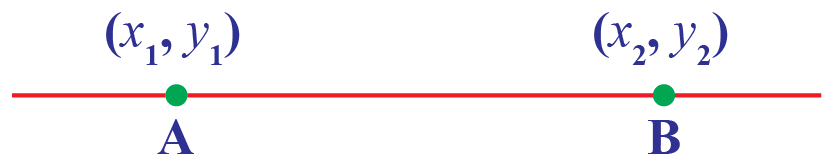A straight line AB is represented by:

 $\overline{\text{AB}}$

While straight lines have no definite beginning or end, they are represented in our day-to-day lives with examples such as railway tracks or the freeway.## Types of Straight Lines

Straight lines are classified on the basis of their alignment.

### Horizontal Lines

The lines which are drawn horizontally are called horizontal lines.

$$\overline{\text{AB}}$$ is a horizontal line.

### Vertical Lines

The lines which are drawn vertically are called vertical lines.

$$\overline{\text{CD}}$$ is a vertical line.### Oblique or Slanting Lines

The lines which are drawn in a slanting position or it is forming some angle other than 0, 90, 180, 270, 360 degrees with the horizontal or vertical lines are called oblique or slanting line.

$$\overline{\text{EF}} \text{ and } \overline{\text{GH}}$$ is a vertical line.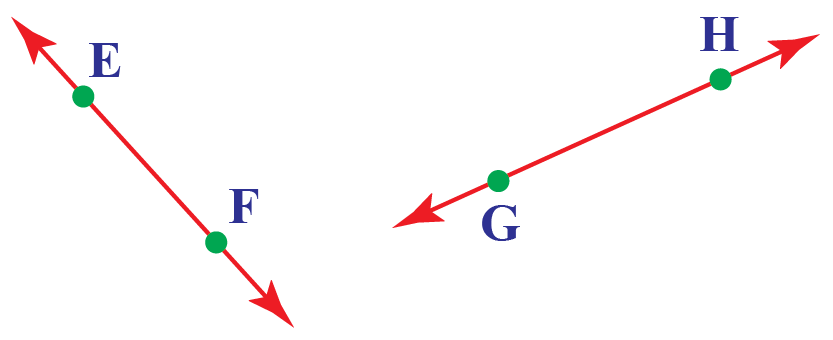## Equation of a Straight Line

A straight line on a cartesian plane can have different representations, some of them are:

### General Equation of a Straight Line

The general equation of a straight line can be given as:

 $ax + by +c = 0$

Where $$a, b, c$$ are constants and $$x, y$$ are variables.

### Slope and Y-intercept Form

A straight line having slope $$m = tan\theta$$ where $$\theta$$ is the angle formed by the line with the positive x-axis, and y-intercept as $$c$$ is given by: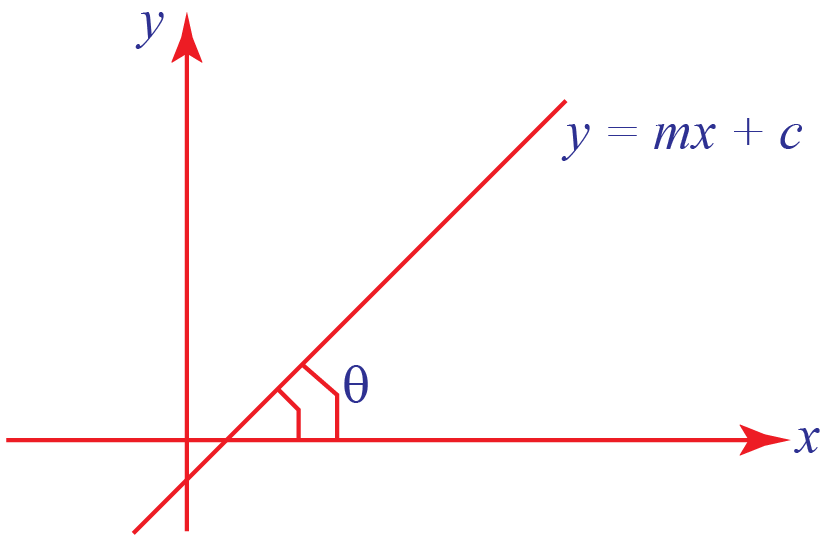$y = mx + c$

### Slope Point Form

A straight line having slope $$m = tan\theta$$ where $$\theta$$ is the angle formed by the line with the positive x-axis, and passing through a point $$(x_1 , y_1)$$ is given by: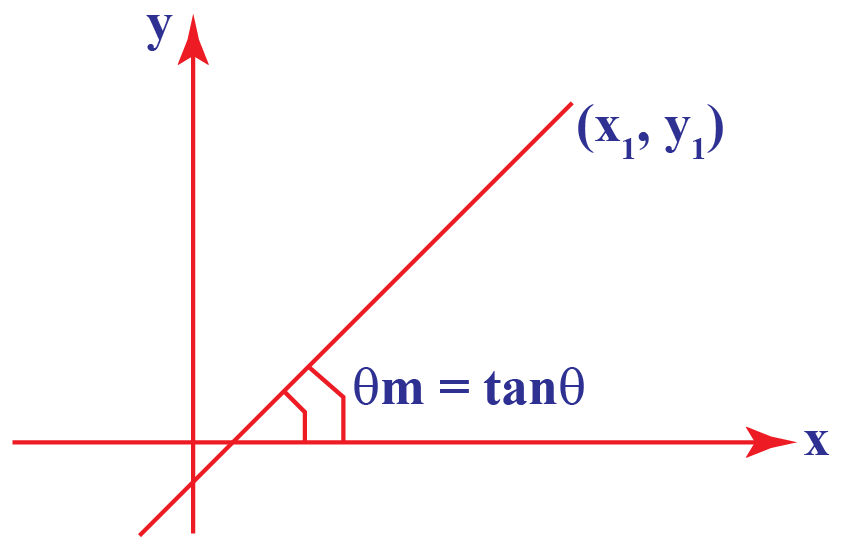$y - y_1 = m(x - x_1)$

### Two Point Form

A straight line passing through points $$(x_1 , y_1)$$ and $$(x_2 , y_2)$$ is given by: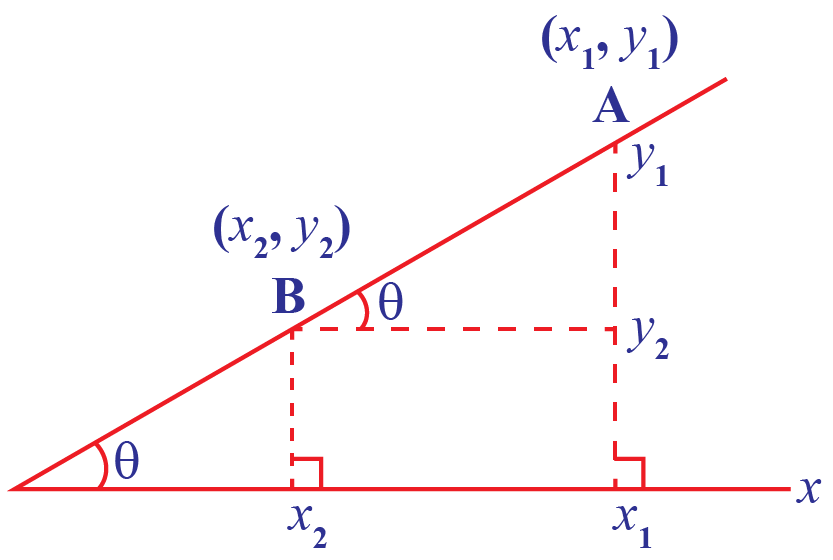$y - y_1 = (\dfrac{y_2 - y_1}{x_2 - x_1})(x - x_1)$

### Intercept Form

A straight line having x-intercept as $$a$$ and y-intercept as $$b$$ as shown in figure below where point A is on x-axis (vertical here) and point B is on y-axis (horizontal here), is given by: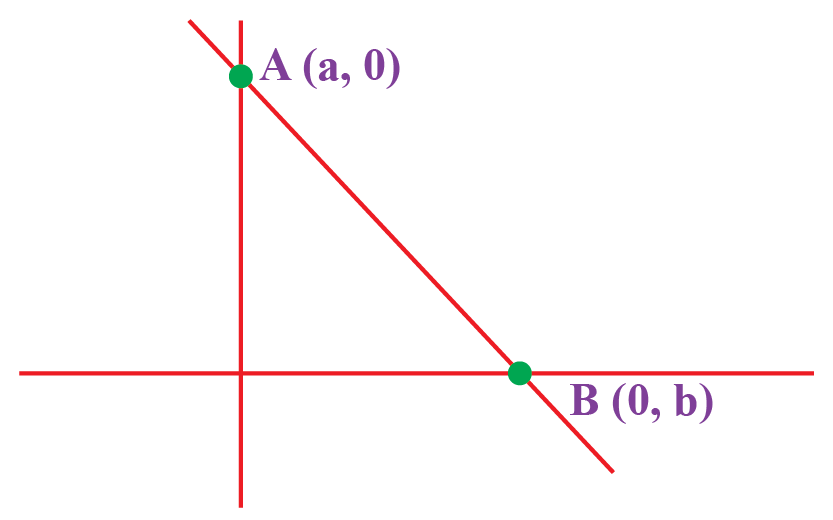$\dfrac{x}{a} + \dfrac{y}{b} = 1$## Straight lines Geometry

There can be so many types of lines that can be formed.

### Line Parallel to Axes

Equation of a line parallel to the axes can be given as:

Line parallel to x-axis

 $y = a$

Where $$a$$ is constant.

Line parallel to y-axis

 $x = b$

Where $$b$$ is constant.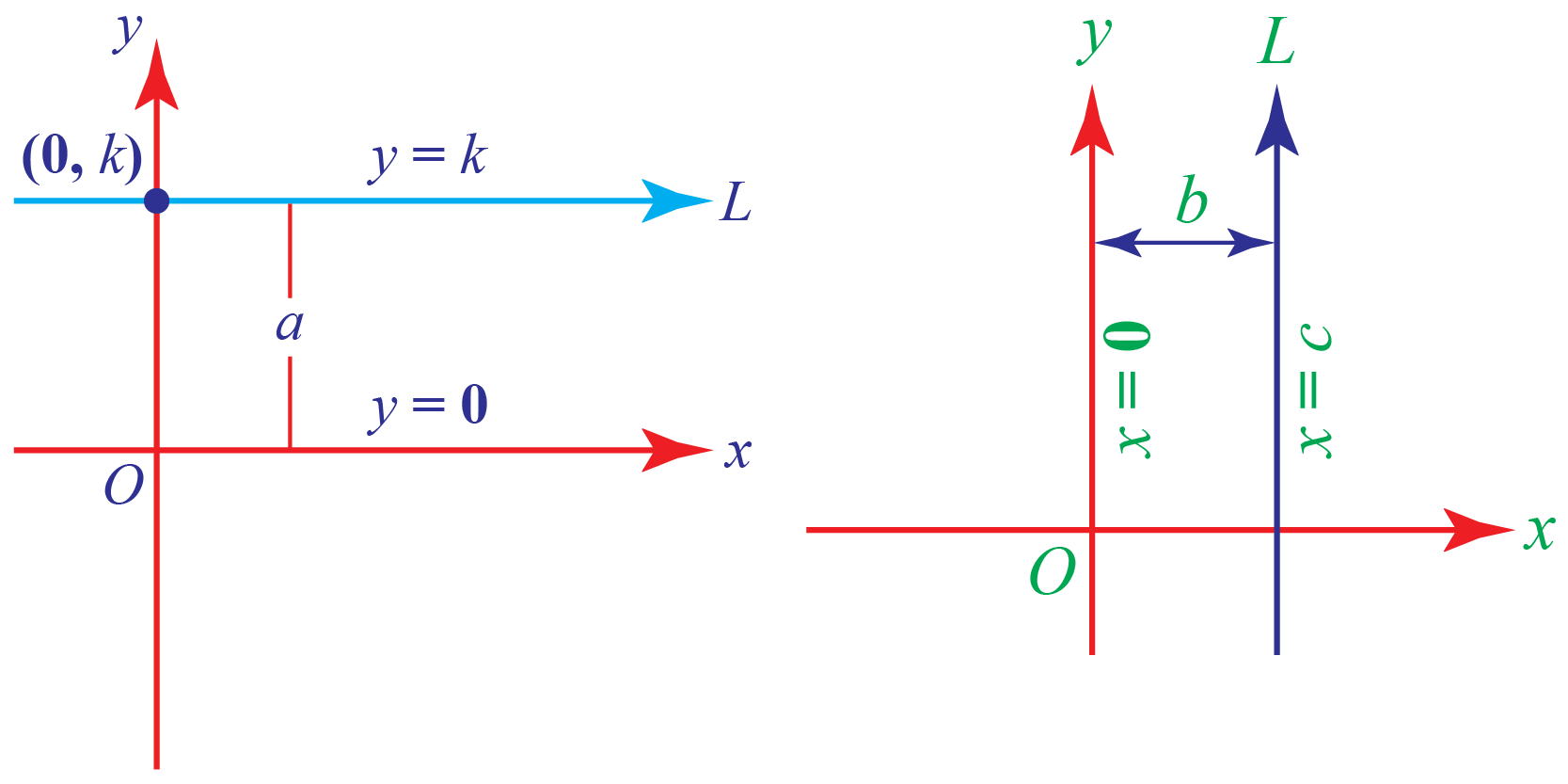### Intersecting Lines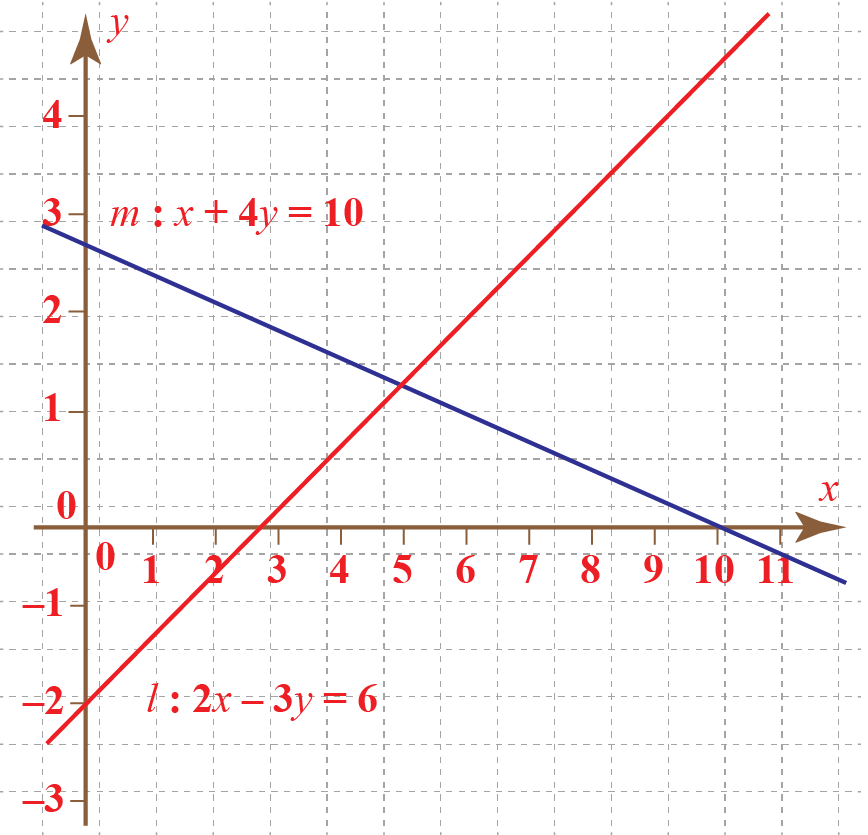If two line $$m: x + 4y = 10$$ and $$n: 2x - 3y = 6$$ intersect at a point $$P (a, b)$$, then coordinates of the point $$P$$ can be calculated by solving the equations of line simulteneously.

\begin{align} x + 4y &= 10\\[0.2cm] 2x - 3y &= 6\\[0.2cm] \end{align}

We will get $$x = \dfrac{30}{11}$$ and $$y = \dfrac{26}{11}$$

So, coordinate of the point $$P$$ is $$(\dfrac{30}{11} , \dfrac{26}{11})$$

### Parallel Lines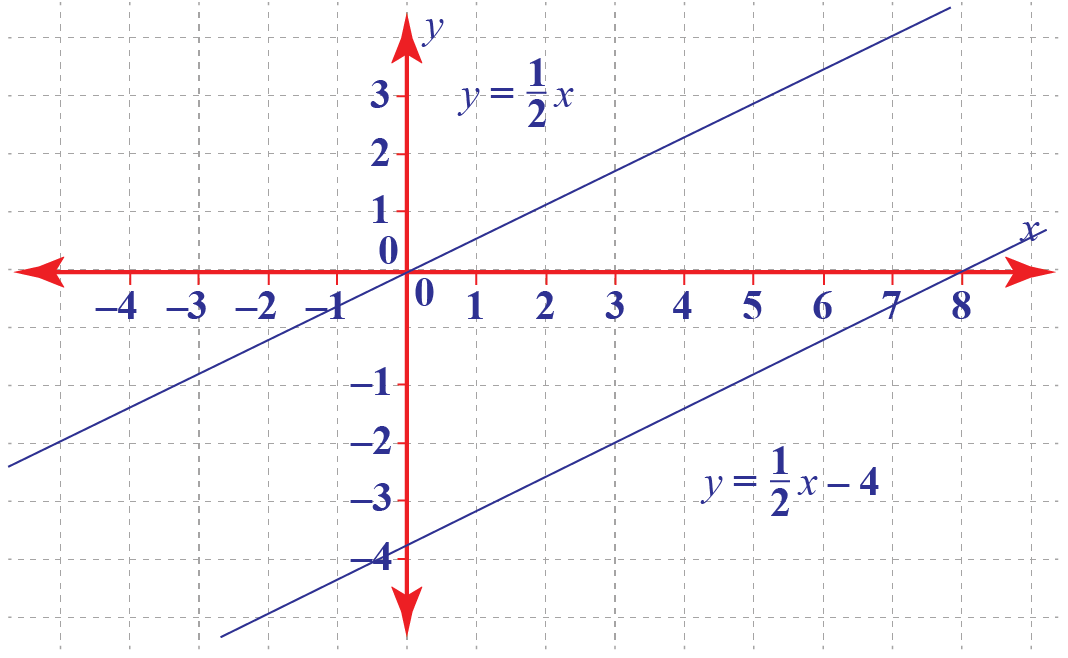If two lines $$y = \dfrac{1}{2}x$$ and $$y = \dfrac{1}{2}x - 4$$ are parallel to each other, then their slopes are equal. Here in this case both the lines have equal slope of $$\dfrac{1}{2}$$.

### Perpendicular Lines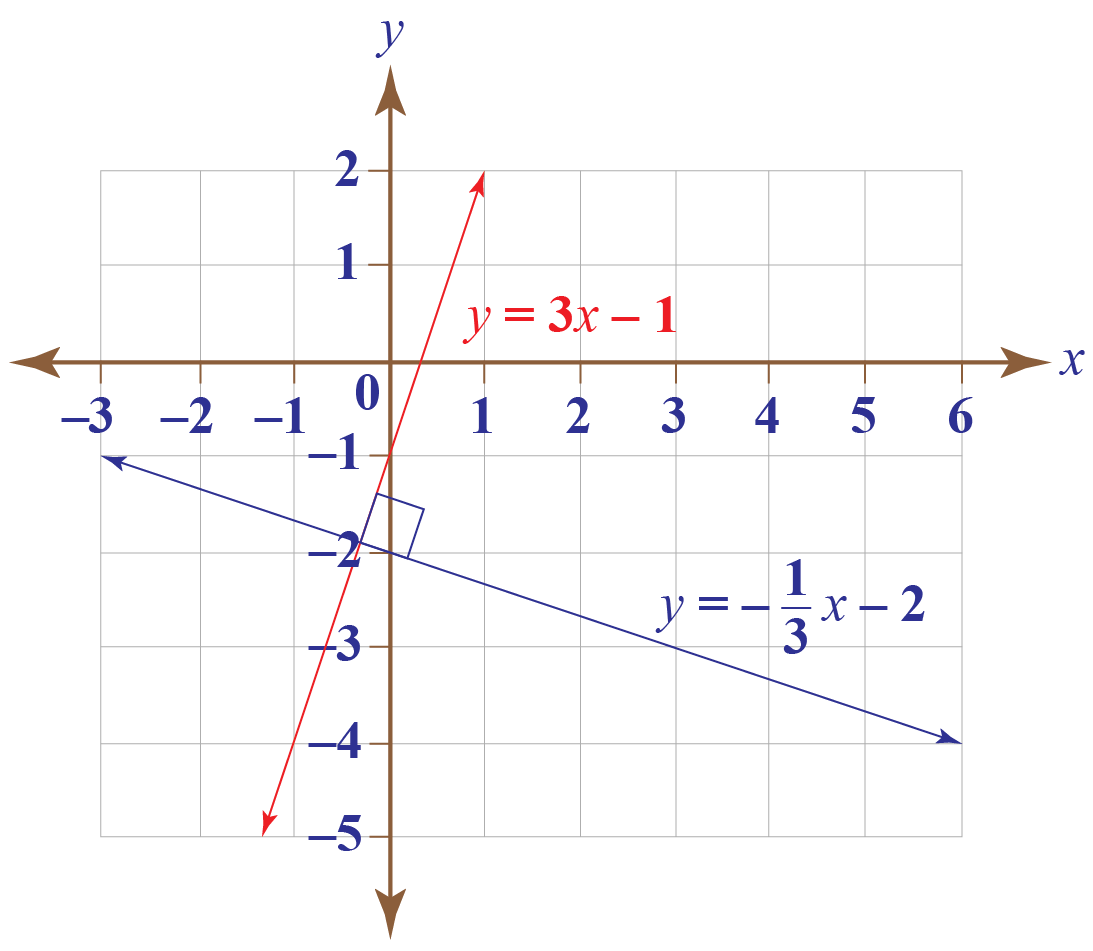If two line $$y = 3x -1$$ and $$y = -\dfrac{1}{3}x - 2$$ are perpendicular to each other, the product of their slopes is $$-1$$.

here in this case:

\begin{align} m_1 &= 3\\[0.2cm] m_2 &= -\dfrac{1}{3}\\[0.2cm] m_1 \times m_2 &= 3 \times -\dfrac{1}{3}\\[0.2cm] m_1 \times m_2 &= -1\\[0.2cm] \end{align}

## Positive or Negative Slope

The angle formed by a line with positive x-axis is the slope of a line, different lines form different angles with the x-axis. Let's see some of the cases.

### Zero Slope

If a line formes $$0^{\circ}$$ angle with the x-axis, the slope of the line is 0.

the slope of a line $$m = tan \theta$$

$$\theta = 0^{\circ}$$ hence $$m = tan 0^{\circ} = 0$$

the line with 0 slope is parallel to x-axis.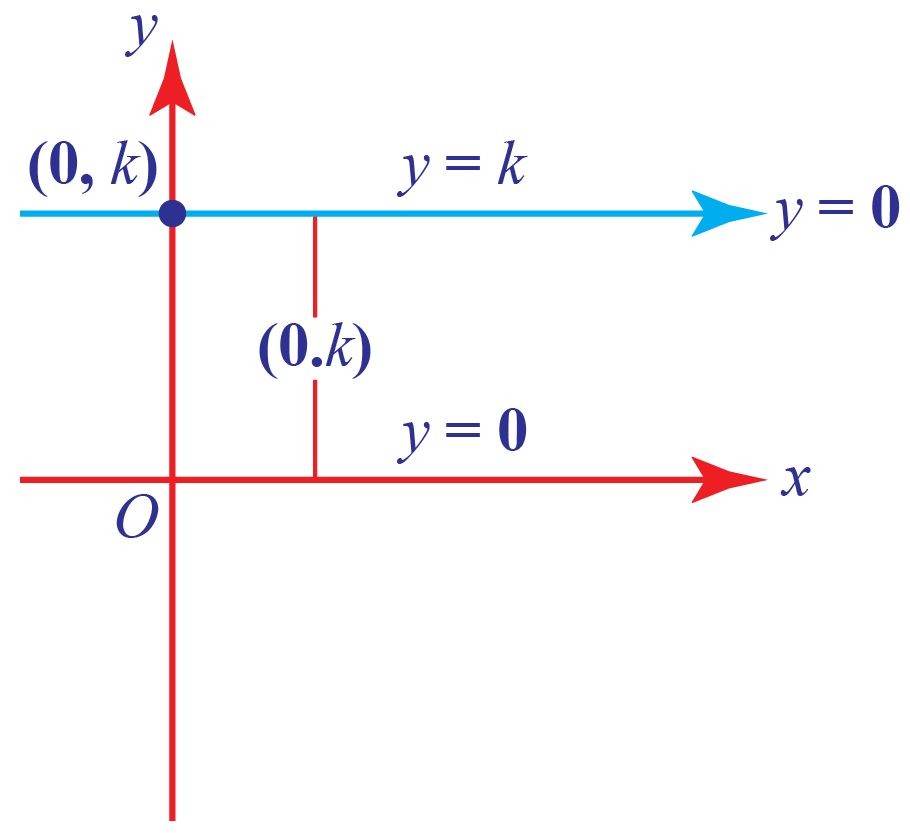### Positive Slope

If a line forms an angle which lies between $$0^{\circ}$$ and $$90^{\circ}$$ with the x-axis, the slope of the line is positive.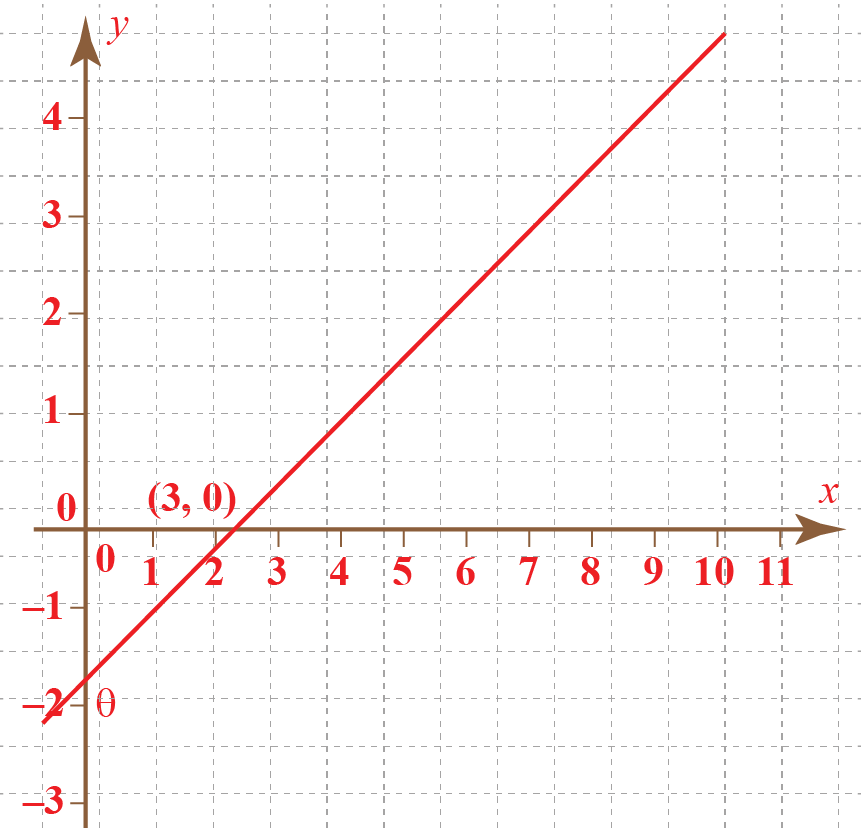### Negative Slope

If a line forms an angle which lies between $$90^{\circ}$$ and $$180^{\circ}$$ with the x-axis, the slope of the line is negative.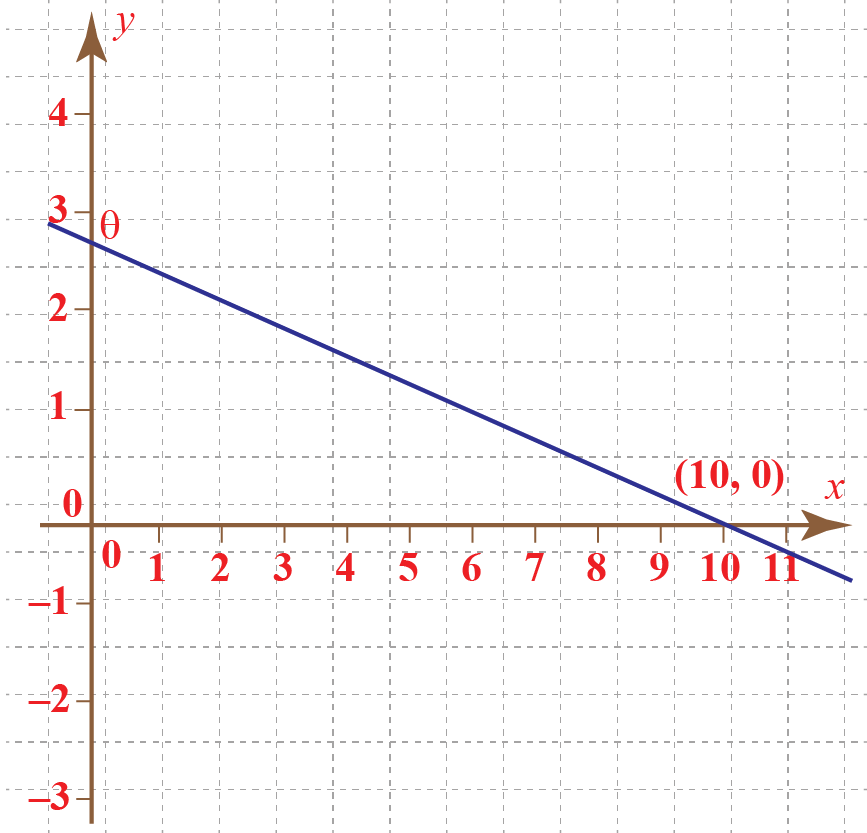### Infinite Slope

If a line formes $$90^{\circ}$$ angle with the x-axis, the slope of the line is not defined or infinite.

the slope of a line $$m = tan \theta$$

$$\theta = 90^{\circ}$$ hence $$m = tan 90^{\circ}$$ which is not defined.

the line with infinite slope is parallel to the y-axis.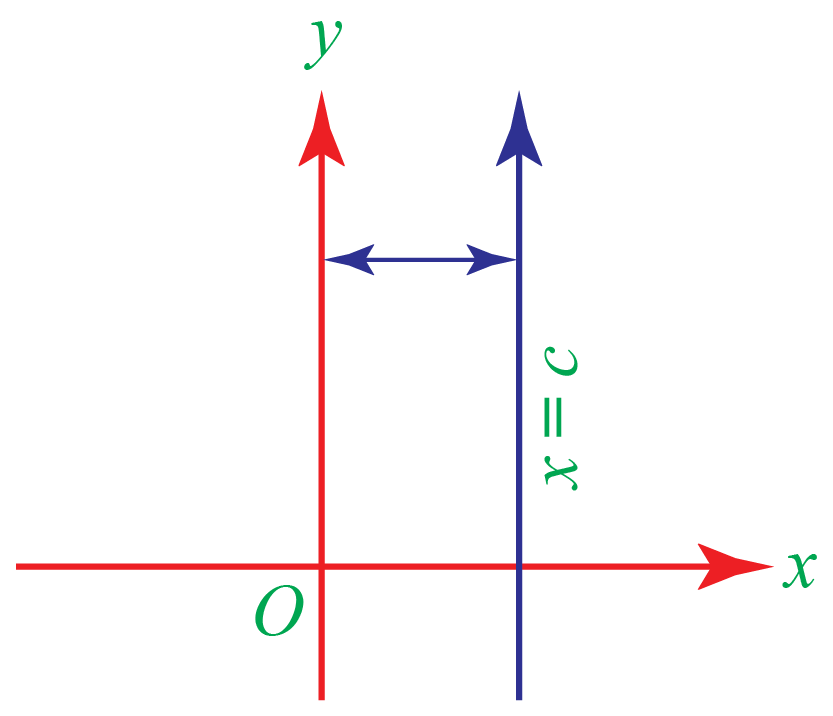## Properties of a Straight Line

1. A straight line has infinite length. We can never calculate the distance between the two extreme points of the line.

2. Whereas what we see around us as lines, for example, the edge of a table, side of rule these all are the examples of Line Segments.

3. A straight line has zero area, zero volume. but it has infinite length.

4. A straight line is a one-dimensional figure, Infine number of lines can pass through a single point, but there is only one unique line which passes through two points.## Identification and Construction of Straight Line

Euclid, the great mathematician, gave some postulates to construct a straight line.

• A straight line segment can be drawn joining any two points.
• Any straight line segment can be extended indefinitely in a straight line.
• Given any straight lines segment, a circle can be drawn having the segment as radius and one endpoint as center.
• All right angles are congruent.
• If two lines are drawn which intersect a third in such a way that the sum of the inner angles on one side is less than two right angles, then the two lines inevitably must intersect each other on that side if extended far enough. This postulate is equivalent to what is known as the parallel postulate.Challenging Questions
• Find the angle between the lines $$l: 4x +5y = 10$$ and $$m: x - 3y = 6$$.
• A tangent dropped on a circle at point (5, 6) if the center of the circle is (1, 2), what is the equation of the tangent.

## Solved Examples

 Example 1

Paul draw a line on a cartesian plane with the equation $$y = 2x - 1$$ and his sister draws the line $$2y = x +1$$, Paul says that the lines intersect in the 2nd quadrant and his sister says that the lines intersect in the 1st quadrant, who is correct.

Solution

Given:
Line drawn by Paul is $$y = 2x - 1$$

his sister drawn the line $$2y = x +1$$

Let's solve these two equations simultaneously to find the point of intersection.
\begin{align} y &= 2x -1\\[0.2cm] 2y &= x +1\\[0.2cm] \end{align}
When se solve these two equations simultaniously we get
$x = 1 \text{ and } y = 1$
Both the line intersect at point $$(1, 1)$$
Point of intersection lies in first quadrant

 $$\therefore$$ Paul's sister is correct
 Example 2

A colony is situated on a cartesian plane, Mathew's house is situated at location $$(4, 3)$$ and Jim's home is located at $$(7, -2)$$ two roads have to be constructed from a square located at $$(3, 2)$$, find out whether these two roads are perpendicular to each other or not (assuming that roads are forming a straight line).

Solution

Let's consider Mathew's house is located at point $$P (4, 3)$$

Jim's house is located at point $$Q (7, -2)$$

Square is situated at point $$R (3, 2)$$

applying the formula to calculate the slope of line between two points
$m = \dfrac{y_2 - y_1}{x_2 - x_1}$
The slope of a line between point P and R is
$m_1 = \dfrac{3 - 2}{4 - 3}$
$m_1 = 1$
The slope of a line between point Q and R is
$m_2 = \dfrac{-2 - 2}{7 - 3}$
$m_2 = \dfrac{-4}{4}$
$m_2 = -1$
If two lines are perpendicular to each other then the product of their slopes is $$-1$$.
$m_1 \times m_2 = 1 \times -1$
$m_1 \times m_2 = -1$

 $$\therefore$$ The roads are perpendicular to each other
 Example 3

A line having x-intercept as $$-1$$ and y-intercept as $$\dfrac{1}{2}$$ another line having a slope equal to 3, what is the angle between these two lines.

Solution

To calculate the angle between two lines we use the formula.
$tan \theta = \dfrac{m_2 - m_1}{1 + m_1 \times m_2}$
Intercept form of a line is
$\dfrac{x}{a} + \dfrac{y}{b} = 1$
$a = -1 \text{ & } b = \dfrac{1}{2}$
Equation of the line is
$\dfrac{x}{-1} + \dfrac{y}{\dfrac{1}{2}} = 1$
$-x + 2y = 1$
Slope of this line $$m_1 = \dfrac{1}{2}$$
Slope of the other line is $$m_2 = 3$$
Using the formula
$tan \theta = \dfrac{3 - \dfrac{1}{2}}{1 + \dfrac{1}{2} \times 3}$
$tan \theta = \dfrac{\dfrac{5}{2}}{\dfrac{5}{2}}$
$tan \theta = 1$
$\theta = 45^{\circ}$

 $$\therefore$$ Angle between the lines is $$45^{\circ}$$

## Interactive Questions

Here are a few activities for you to practice.

## Let's Summarize

The mini-lesson targeted in the fascinating concept of straight lines. The math journey around straight lines starts with what a student already knows, and goes on to creatively crafting a fresh concept in the young minds. Done in a way that not only it is relatable and easy to grasp, but also will stay with them forever. Here lies the magic with Cuemath.

At Cuemath, our team of math experts is dedicated to making learning fun for our favorite readers, the students!

Through an interactive and engaging learning-teaching-learning approach, the teachers explore all angles of a topic.

Be it worksheets, online classes, doubt sessions, or any other form of relation, it’s the logical thinking and smart learning approach that we at Cuemath believe in.

## 1. What is a line in maths?

A line is a one-dimensional figure formed when we connect two distinct points with minimum distance between the points.

## 2. What is a point?

A point is a dot in a plane with zero length, zero area, and zero volume, a point has no dimensions.

## 3. What are line segments and rays?

Line segment is a part of a line that has fixed ends and its length can be calculated by using the measurement techniques.
Rays are also the part of a line but it has only one fixed end and the other end of a ray is considered to be at infinity, sun rays are the perfect example of rays.

More Important Topics
Numbers
Algebra
Geometry
Measurement
Money
Data
Trigonometry
Calculus